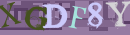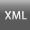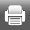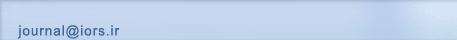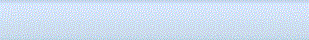[Home ] [Archive]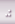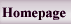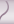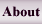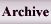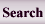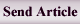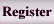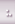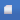Volume 2, Issue 1 (vol 2. No 1 2010)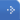IJOR 2010, 2(1): 77-88 Back to browse issues page
Close interval approximation of piecewise quadratic fuzzy numbers for fuzzy fractional program
Jain
Abstract:   (24396 Views)

The fuzzy approach has undergone a profound structural transformation in the past few decades. Numerous studies have been undertaken to explain fuzzy approach for linear and nonlinear programs. While, the findings in earlier studies have been conflicting, recent studies of competitive situations indicate that fractional programming problem has a positive impact on comparative scenario. We propose one of the best interval approximations, close interval approximation of piecewise quadratic fuzzy numbers for solving fuzzy number fractional programming problem without converting it to a crisp problem. A new form of simplex method is introduced here for solving fuzzy number fractional programming problem using fuzzy arithmetic. The fuzzy analogue of some important theorems of fuzzy fractional programming problem proved. A fuzzy fractional programming problem is worked out as an example to illustrate the proposed method.

Keywords: Piecewise quadratic fuzzy number, Fuzzy fractional programming problem Fuzzy linear equations, Close interval approximation.